# vs.eyeandcontacts.com

## Chapter 4 Practical Geometry Exercise 4.1

Question 1: Construct the following quadrilaterals.
AB = 4.5 cm
BC = 5.5 cm
CD = 4 cm
AC = 7 cm
JU = 3.5 cm
UM = 4 cm
MP = 5 cm
PJ = 4.5 cm
PU = 6.5 cm
iii) Parallelogram MORE
OR = 6 cm
RE = 4.5 cm
EO = 7.5 cm
iv) Rhombus BEST
BE = 4.5 cm
ET = 6 cm

i)
Given
AB = 4.5 cm
BC = 5.5 cm
CD = 4 cm
AC = 7 cm

Rough figure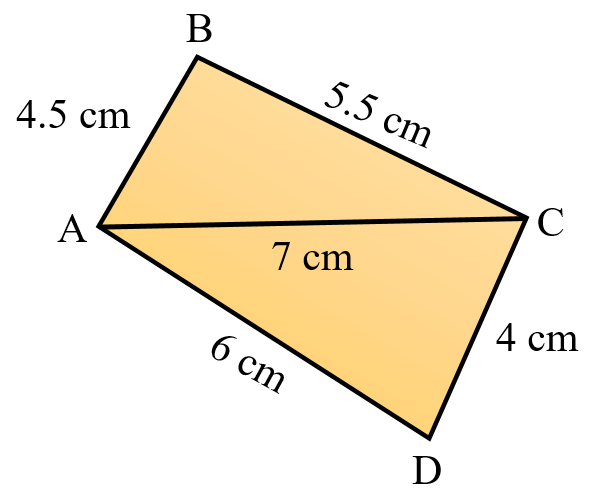Actual figure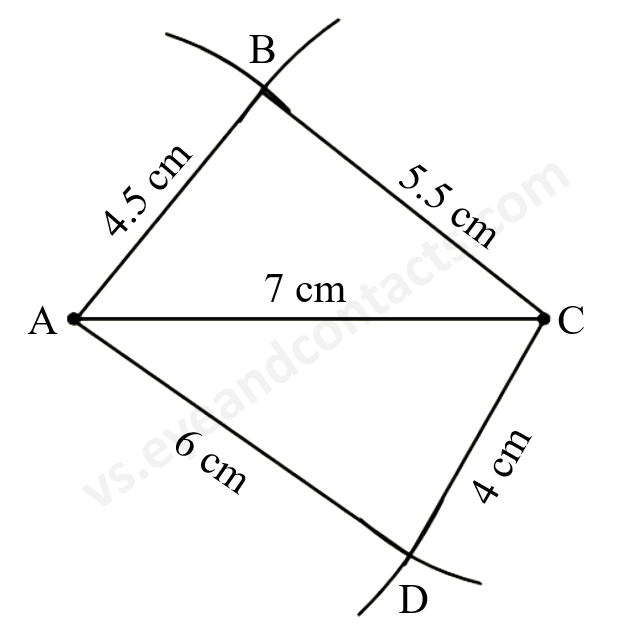Steps of Construction
1. Draw line segment AC = 7 cm.
2. With A as centre, radius 4.5 cm draw an arc.
3. With C as centre, radius 5.5 cm draw an arc to cut previous arc at B.
4. With A as centre and radius 6 cm draw an arc.
5. With C as centre and radius 4 cm draw an arc to cut previous arc at D.
6. Join, AB, BC, AD, CD.
7. ABCD is the required quadrilateral.

ii)
Given
JU = 3.5 cm
UM = 4 cm
MP = 5 cm
PJ = 4.5 cm
PU = 6.5 cm

Rough figure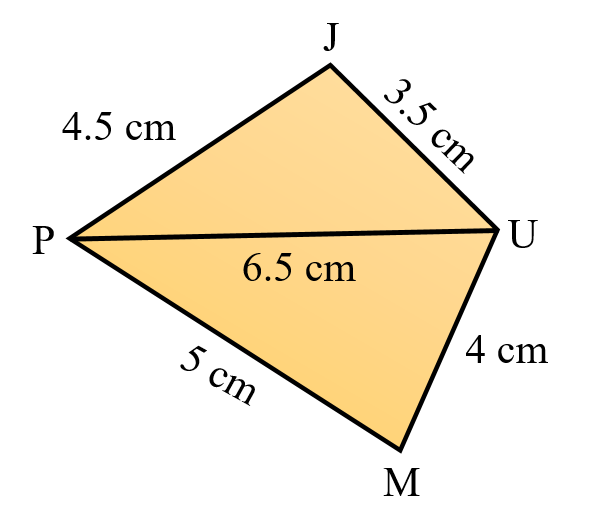Actual figure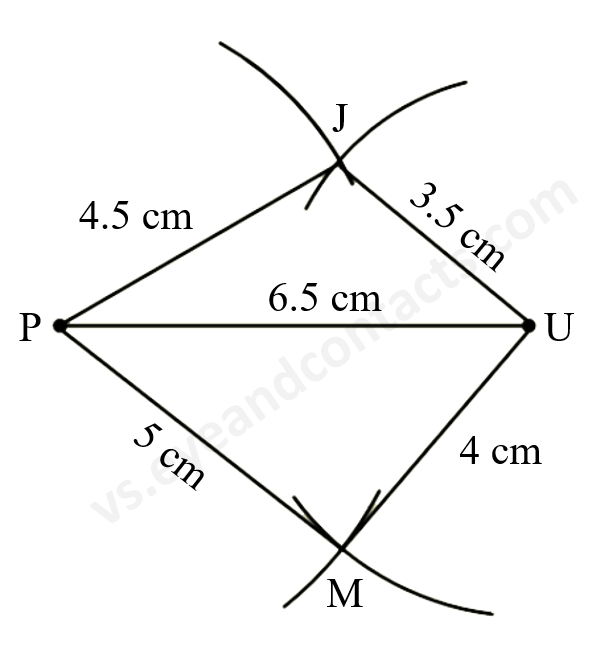Steps of Construction
1. Draw line segment PU = 6.5 cm.
2. With P as centre, radius 4.5 cm draw an arc.
3. With U as centre, radius 3.5 cm draw an arc to cut the previous arc at J.
4. With P as centre, radius 5 cm draw an arc.
5. With U as centre, radius 4 cm, draw an arc to cut the previous arc at M.
6. Join JP, JU, PM, UM.
7. JUMP is the required quadrilateral.

iii)
Given
Parallelogram MORE
OR = 6 cm
RE = 4.5 cm
EO = 7.5 cm

Rough figureActual figure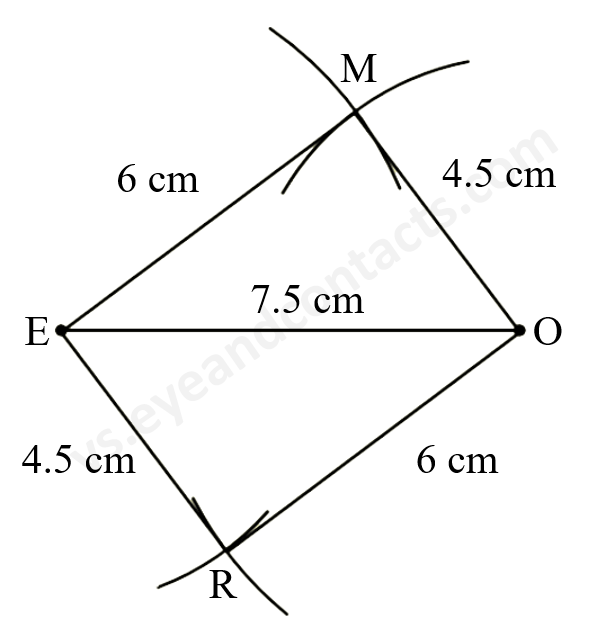Steps of Construction
1. Draw line segment EO = 7.5 cm.
2. With E as centre, radius 6 cm draw an arc.
3. With O as centre, radius 4.5 cm draw another arc to cut the previous arc at M.
4. With E as centre, radius 4.5 cm draw an arc.
5. With O as centre, radius 6 cm draw an arc to cut the previous arc at R.
6. Join ME, MO, ER, OR.
7. MORE is the required parallelogram.

iv)
Given
Rhombus BEST
BE = 4.5 cm
ET = 6 cm

Rough figureActual figure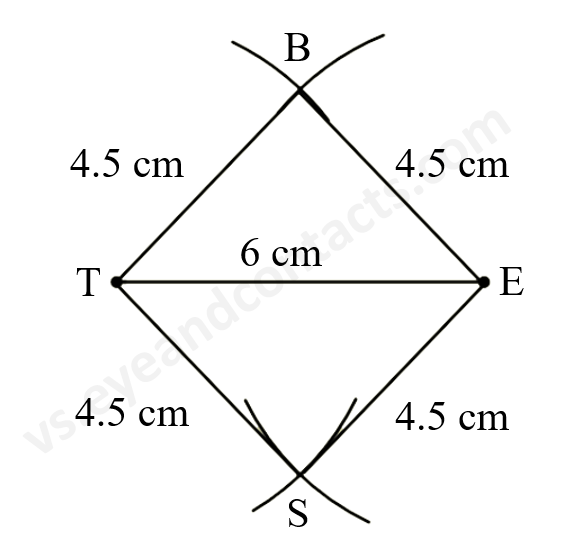Steps of Construction
1. Draw line segment TE = 6 cm.
2. With T as centre, radius 4.5 cm draw an arc.
3. With E as centre, radius 4.5 cm draw an arc to cut previous arc at B.
4. With T as centre, radius 4.5 cm draw an arc.
5. With E as centre, radius 4.5 cm draw an arc to cut the previous arc at S.
6. Join BT, BE, TS, ES.
7. BEST is the required rhombus.